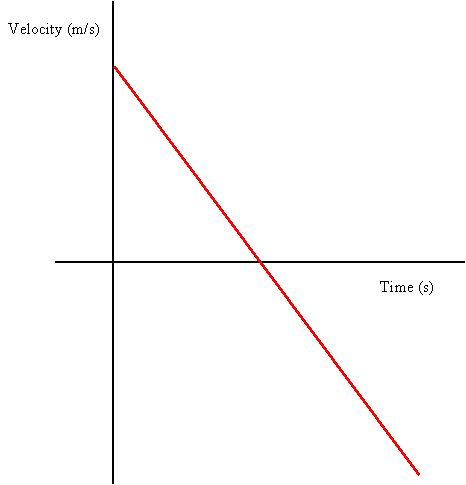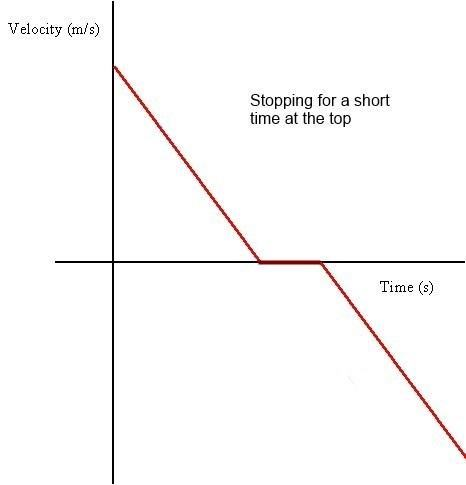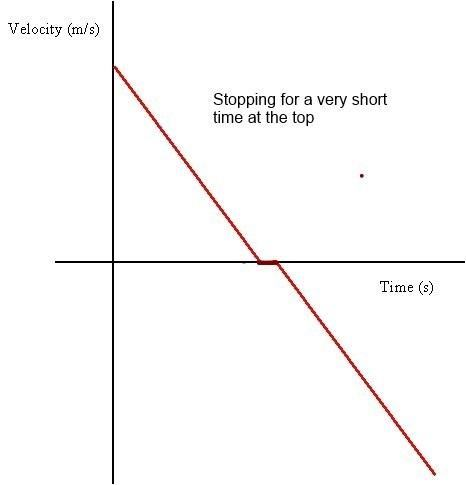# Velocity graph, a ball is thrown upward

Not for a ball thrown up. In a different scenario you might have zero slope somewhere. For example, the velocity function of an elevator. Also, if you include air resistance, the velocity graph for the ball will become non-linear, but you still won't get zero slope at the turnaround point, because the drag is zero there. Only if the ball would approach terminal velocity when coming down, the graph would approach the horizontal.fI don't get it, why is the graph always linear? Shouldn't its slope be zero when the ball is at the top and stops there for a moment?
The way it is "explained" is that it's at the top for instantaneous moment. Okay, but, if let's say, ball was going upward for a 5 sec, then stopped for 1 second and went downwards. Wouldn't you agree with me, that if we were to record the ball's motion, and compared its velocity between 3 and 4 seconds, the difference would be much higher than if we compared velocity between 5 and 6 seconds (when the ball's velocity isn't changing, it simply hangs in the air, therefore there would be no difference in velocity between 5s and 6s)?? If so, then it should be reflected on the graph, considering that the scale is fixed.

When the ball is at the top and stops for a moment, the velocity of the ball is zero. This is represented as the point where the velocity graph crosses the time axis.

The slope of the velocity graph, however, describes how the velocity is changing, and has nothing to do with if the ball is stationary or moving. Zero velocity means the velocity graph is at zero; it does not mean the graph's slope is zero.

The slope of the position graph at this moment would indeed be zero, but not that of the velocity graph.

then stopped for 1 second
That is not what happens in free fall.

it should be reflected on the graph
Not for a ball thrown up. In a different scenario you might have zero slope somewhere. For example, the velocity function of an elevator. Also, if you include air resistance, the velocity graph for the ball will become non-linear, but you still won't get zero slope at the turnaround point, because the drag is zero there. Only if the ball would approach terminal velocity when coming down, the graph would approach the horizontal.

Last edited:
Shouldn't its slope be zero when the ball is at the top and stops there for a moment?

The ball doesn't literally stop. It appears to be stationary for a fraction of a second when the direction of velocity changes.

The velocity axis (y-axis) shows whether the ball in going up or down. (positive : ball going up, negative : ball going down)

The moment when the direction of velocity changes is the one where the magnitude of velocity is zero (where it cuts the x-axis)I don't get it, why is the graph always linear?
v(t) = v0 - g⋅t
If acceleration g is constant, v(t) is a linear function.

This graph linear because only acceration hapening here is gravitical (g) . acceleration. And this ball drain its velocity with (g) and it stopped its motion when its velocity=0ms-1. Instantly it change its motion to earth. This insident happening in very small time so its not important. But after this its velocity gets (-) mark because velocity's derection changed.

The ball doesn't literally stop.

The ball does literally stop. "Stop" means its velocity reaches zero. It does not mean it stays there for any particular length of time. But it definitely reaches velocity zero.

The ball does literally stop. "Stop" means its velocity reaches zero. It does not mean it stays there for any particular length of time. But it definitely reaches velocity zero.

Yes. Agreed. But dramadeaur was a certain amount of time for which the velocity would remain 0. Which would mean the graph would be horizontal at v = 0 for a small time. But that isn't the case. Just wanted to say that.

I don't get it, why is the graph always linear?
Because the acceleration due to gravity is constant.

Shouldn't its slope be zero when the ball is at the top and stops there for a moment?

Zero slope would imply constant velocity or zero acceleration. When would the velocity be constant? The acceleration due to gravity is never zero.

At the top the velocity is zero. This is shown on the graph when the velocity crosses the time axis and changes from +ve to -ve.

The way it is "explained" is that it's at the top for instantaneous moment. Okay, but, if let's say, ball was going upward for a 5 sec, then stopped for 1 second and went downwards...

Ok let's suppose that the ball did stop at the top for a short time (perhaps someone catches it and then drops it). The graph would look like the one below.If the ball stopped for an even shorter time it would look like the one below.If you keep making the time that it's stationary shorter and shorter you end up with your original drawing.

Last edited:
Yes. Agreed. But dramadeaur was a certain amount of time for which the velocity would remain 0. Which would mean the graph would be horizontal at v = 0 for a small time. But that isn't the case. Just wanted to say that.

View attachment 78170

If the ball stopped for an even shorter time it would look like the one below.

View attachment 78171

If you keep making the time that it's stationary shorter and shorter you end up with your original drawing.

If the ball was going upwards for 6 seconds (at 6th second it reaches the top) Does it mean that the change in velocity between 4-5 seconds would be the same as the change in velocity between 6-7 seconds?

Does it mean that the change in velocity between 4-5 seconds would be the same as the change in velocity between 6-7 seconds?
Yes, as already sated multiple times here, acceleration due to gravity is constant (on such small scales).

I don't really understand the bottom part of the graph. As what you guys told here, i understand the upper part of the graph. The upper graph of the graph indicates that the ball is thrown upwards and reached the maximum height.But then how about the lower part?? is the ball bouncing upwards again or the ball is just collapsed??

I don't really understand the bottom part of the graph. As what you guys told here, i understand the upper part of the graph. The upper graph of the graph indicates that the ball is thrown upwards and reached the maximum height.But then how about the lower part?? is the ball bouncing upwards again or the ball is just collapsed??
Note that it is a graph of VELOCITY not SPEED. The graph assumes that up is positive. At the start the ball is going up with some initial velocity. That velocity reduces until it reaches zero at the top. The ball then falls. On the way down the velocity is therefore negative.

At some point it hits the ground with the same SPEED that it was launched with but the VELOCITY will be negative.

•sophiecentaur
Note that it is a graph of VELOCITY not SPEED. The graph assumes that up is positive. At the start the ball is going up with some initial velocity. That velocity reduces until it reaches zero at the top. The ball then falls. On the way down the velocity is therefore negative.

At some point it hits the ground with the same SPEED that it was launched with but the VELOCITY will be negative.
Interpreting graphs is not an innate skill. A descending line implies downward motion to someone who does't automatically read the labels on axes. I defy anyone, even someone with years of experience of using and interpreting graphs, to claim that, even now, they always get it right first time. Using the Area under a graph to show a Distance travelled, is another real hurdle for the uninitiated.
Give students the task of drawing graphs of position, velocity and acceleration for a bouncing superball. You are guaranteed to get some bizarre results.

Note that it is a graph of VELOCITY not SPEED. The graph assumes that up is positive. At the start the ball is going up with some initial velocity. That velocity reduces until it reaches zero at the top. The ball then falls. On the way down the velocity is therefore negative.

At some point it hits the ground with the same SPEED that it was launched with but the VELOCITY will be negative.
Oh I got it! Thank you for spending your time here~